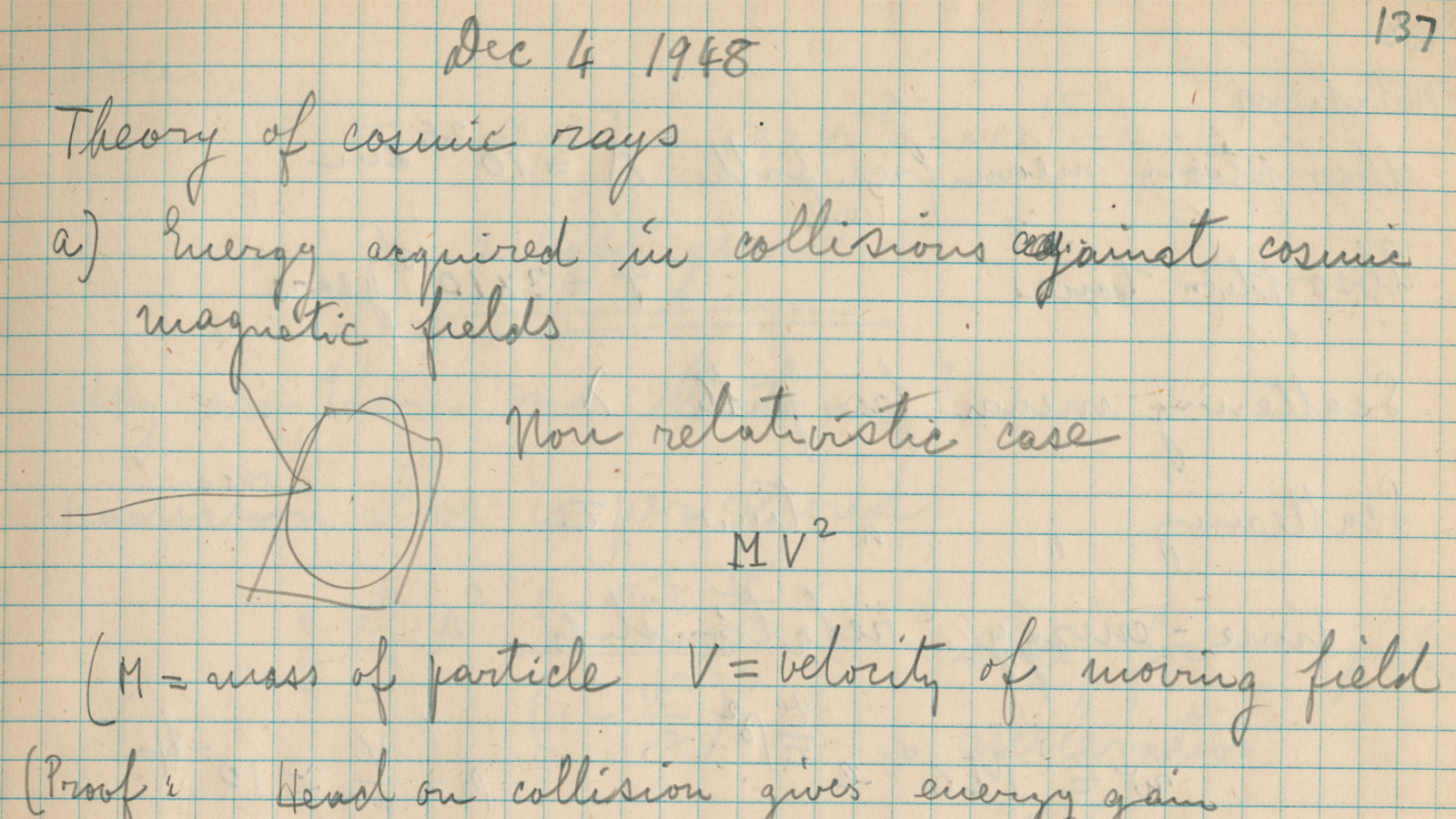A joint Fermilab/SLAC publicationUniversity of Chicago Library

# Cosmic rays

11/21/12

Enrico Fermi's notebook of December 1948 contains four pages that represent the genesis of his theory of cosmic rays, particles that can hit the Earth's atmosphere at high speeds. In these pages, he worked out the acceleration of cosmic rays due to a series of collisions with magnetic clouds moving through the universe, a process later named Fermi acceleration.

Enrico Fermi's notebook of December 1948 contains four pages that represent the genesis of his theory of cosmic rays, particles that can hit the Earth's atmosphere at high speeds. In these pages, he worked out the acceleration of cosmic rays due to a series of collisions with magnetic clouds moving through the universe, a process later named Fermi acceleration.

Fermi's non-relativistic calculation on this page shows that a cosmic-ray particle gains an energy of MV2 per collision, where M is the mass of the cosmic-ray particle and V is the velocity of the cloud. From this he concluded—in typical Fermi style—that the relativistic energy gain is w β2, where w is the total energy of the particle, and β is the ratio of V to the speed of light. (As an afterthought, Fermi carried out the exact calculation on page 140, which yields 4/3 w β2.) Fermi went on to calculate the average distance between collisions with clouds as 2 light years, a reasonable value for magnetic clouds in a galaxy.

Within four weeks of writing these notes, Fermi submitted a paper titled "On the Origin of the Cosmic Radiation" to the journal Physical Review, which published the article in its April 15, 1949, issue.

Today, Fermi acceleration is the accepted mechanism by which cosmic-ray particles gain energy. We now know that the energy gain comes from collisions with turbulent magnetic fields on either side of a shock wave generated by a supernova, which yields an energy gain per collision proportional to β.University of Chicago Library